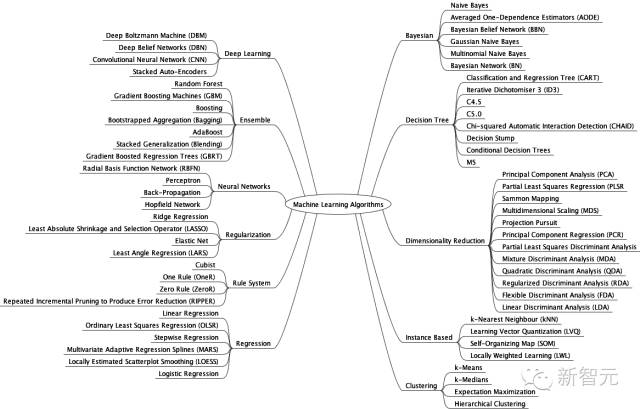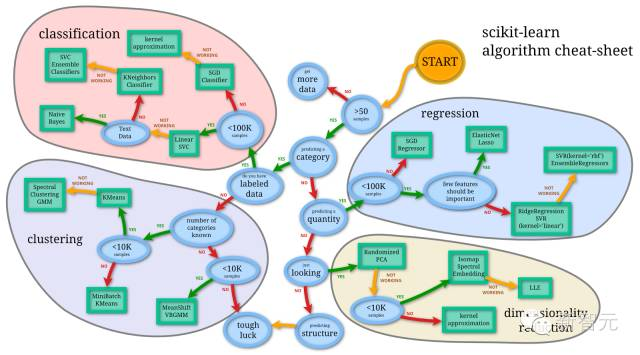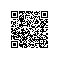# 【干货】机器学习常用 35 大算法盘点（附思维导图）• 普通最小二乘回归（OLSR）

• 线性回归

• 逻辑回归

• 逐步回归

• 多元自适应回归样条法（MARS）

• 局部估计平滑散点图（LOESS）

• k-邻近算法（kNN）

• 学习矢量量化算法（LVQ）

• 自组织映射算法（SOM）

• 局部加权学习算法（LWL）

• 岭回归

• LASSO 算法

• Elastic Net

• 最小角回归算法（LARS）

• 分类和回归树（CART）

• ID3 算法

• C4.5 算法和 C5.0 算法（它们是一种算法的两种不同版本）

• CHAID 算法

• 单层决策树

• M5 算法

• 条件决策树

• 朴素贝叶斯算法

• 高斯朴素贝叶斯算法

• 多项式朴素贝叶斯算法

• AODE 算法

• 贝叶斯信念网络（BBN）

• 贝叶斯网络（BN）

• K-均值

• K-中位数

• EM 算法

• 分层聚类算法

• Apriori 算法

• Eclat 算法

• 感知机

• 反向传播算法（BP 神经网络）

• Hopfield网络

• 径向基函数网络（RBFN）

• 深度玻尔兹曼机（DBM）

• 深度信念网络（DBN）

• 卷积神经网络（CNN）

• 栈式自编码算法（Stacked Auto-Encoder）

• 主成分分析法（PCA）

• 主成分回归（PCR）

• 偏最小二乘回归（PLSR）

• 萨蒙映射

• 多维尺度分析（MDS）

• 投影寻踪法（PP）

• 线性判别分析（LDA）

• 混合判别分析（MDA）

• 二次判别分析（QDA）

• 灵活判别分析（Flexible Discriminant Analysis，FDA）

• Boosting

• Bagging

• 堆叠泛化（混合）

• GBM 算法

• GBRT 算法

• 随机森林

• 特征选择算法

• Algorithm accuracy evaluation

• Performance measures